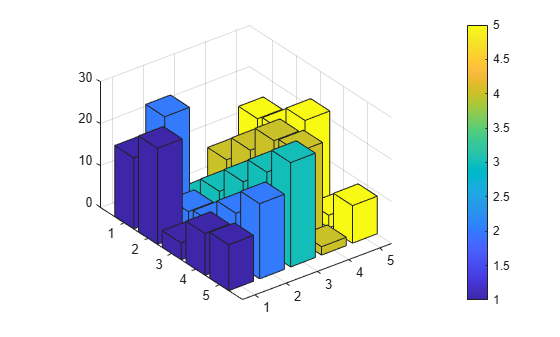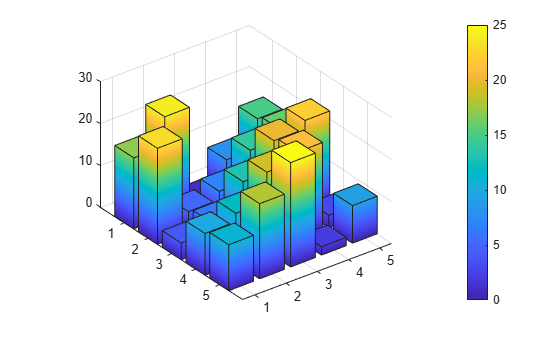Main Content

# Color 3-D Bars by Height

This example shows how to modify a 3-D bar plot by coloring each bar according to its height.

Create a 3-D bar graph of data from the `magic` function. Return the surface objects used to create the bar graph in array `b`. Add a colorbar to the graph.

```Z = magic(5); b = bar3(Z); colorbar```For each surface object, get the array of z-coordinates from the `ZData` property. Use the array to set the `CData` property, which defines the vertex colors. Interpolate the face colors by setting the `FaceColor` properties of the surface objects to `'interp'`. Use dot notation to query and set properties.

```for k = 1:length(b) zdata = b(k).ZData; b(k).CData = zdata; b(k).FaceColor = 'interp'; end```The height of each bar determines its color. You can estimate the bar heights by comparing the bar colors to the colorbar.

Download ebook